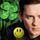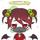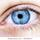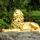## Social Question# Can you solve this simple algebra problem?

Asked by LostInParadise (31601) December 1st, 2021

I wonder how many high school students could solve this. The answer is so simple that it seems that it should be intuitive, but I can’t solve it without doing a little bit of basic algebra.

x = 10(y-x). Solve for y in terms of (y – x).

Observing members: 0Composing members: 0I could not do it in my head. I had to use simple algebra.

LuckyGuy (43566)“Great Answer” (3) Flag as…y=x/10 + x

ragingloli (51727)“Great Answer” (2) Flag as…Loli, you are very close. Substitute for x in your equation to get y in terms of y-x.

LostInParadise (31601)“Great Answer” (0) Flag as…Then I do not understand what “solve for y in terms of y-x” means.

ragingloli (51727)“Great Answer” (0) Flag as…y=f(y-x)

LostInParadise (31601)“Great Answer” (0) Flag as…I love the smell of Algebra in the morning. It smells like victory.

Forever_Free (8244)“Great Answer” (1) Flag as…I still do not know what that means.

ragingloli (51727)“Great Answer” (0) Flag as…x=10(y-x) expresses x in terms of y-x. x is 10 times greater than the quantity (y-x). If we designate y-x as d then we can say that x =10d. There is a similar equation that gives y in terms of (y-x).

LostInParadise (31601)“Great Answer” (0) Flag as…Is that what you are looking for?
x=10(y-x)
x=10y-10x
x=y+9y-10x
0=y+9y-9x
y=9y-9x
y=9(y-x)

ragingloli (51727)“Great Answer” (0) Flag as…Hang on, no, that’s wrong.

ragingloli (51727)“Great Answer” (0) Flag as…x=10(y-x)
x=10y-10x
x=y+9y-10x
0=y+9y-11x
y=9y-11x
y=9y-9x-2x
y+2x=9(y-x)
y=9(y-x)+2x

ragingloli (51727)“Great Answer” (0) Flag as…Your original was very close: y=x/10 + x. Substitute 10(y-x) for the two x values.

Another approach starts out y= x + (y-x). Do you see how a single substitution gives us what we want?

LostInParadise (31601)“Great Answer” (0) Flag as…If I substitute the x, I end up with
x=10(y-x)
10(y-x)=10(y-(10(y-x)))
y-x=y-10y-10x
y-x=-9y-10x
y=-9y-9x
y=-9(y+x)

your second one instantly resolves to y=y

ragingloli (51727)“Great Answer” (0) Flag as…y = x + (y-x) = 10(y-x) + (y-x) = 11(y-x)

From your original, y =x/10 + x = 10(y-x)/10 + 10(y-x) = 11(y-x)

LostInParadise (31601)“Great Answer” (1) Flag as…I would tend to prefer to solve for y in terms of x:
x = 10(y-x)
x/10 = y – x
y = x/10 + x
y = 1.1x

But in terms of (y – x), I think it is asking us to do something like:
x = 10(y – x)
y = 10(y – x) + y – x
y = 11(y – x)

Zaku (30163)“Great Answer” (1) Flag as…